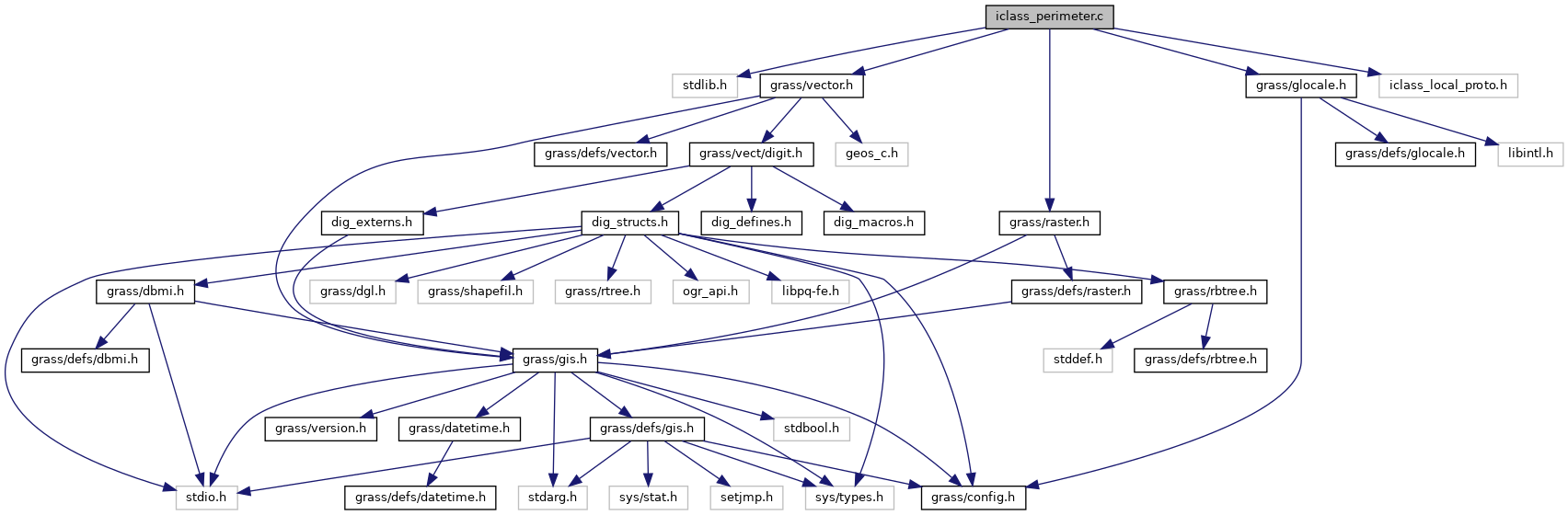GRASS GIS 7 Programmer's Manual  7.9.dev(2021)-e5379bbd7
iclass_perimeter.c File Reference

Imagery library - functions for wx.iclass. More...

`#include <stdlib.h>`
`#include <grass/vector.h>`
`#include <grass/raster.h>`
`#include <grass/glocale.h>`
`#include "iclass_local_proto.h"`
Include dependency graph for iclass_perimeter.c:Go to the source code of this file.

## Macros

#define extrema(x, y, z)   (((x<y)&&(z<y))||((x>y)&&(z>y)))

#define non_extrema(x, y, z)   (((x<y)&&(y<z))||((x>y)&&(y>z)))

## Functions

int vector2perimeters (struct Map_info *Map, const char *layer_name, int category, IClass_perimeter_list *perimeters, struct Cell_head *band_region)
Creates perimeters from vector areas of given category. More...

void free_perimeters (IClass_perimeter_list *perimeters)
Frees all perimeters in list of perimeters. More...

int make_perimeter (struct line_pnts *points, IClass_perimeter *perimeter, struct Cell_head *band_region)
Creates one perimeter from vector area. More...

int edge2perimeter (IClass_perimeter *perimeter, int x0, int y0, int x1, int y1)
Converts edge to cells. More...

void perimeter_add_point (IClass_perimeter *perimeter, int x, int y)
Adds point to perimeter. More...

int edge_order (const void *aa, const void *bb)
Determines points order during sorting. More...

## Detailed Description

Imagery library - functions for wx.iclass.

Computation based on training areas for supervised classification. Based on i.class module (GRASS 6).

Vector map with training areas is used to determine corresponding cells by computing cells on area perimeter.

Copyright (C) 1999-2007, 2011 by the GRASS Development Team

This program is free software under the GNU General Public License (>=v2). Read the file COPYING that comes with GRASS for details.

Definition in file iclass_perimeter.c.

## ◆ extrema

 #define extrema ( x, y, z ) (((xy)&&(z>y)))

Definition at line 37 of file iclass_perimeter.c.

## ◆ non_extrema

 #define non_extrema ( x, y, z ) (((xy)&&(y>z)))

Definition at line 38 of file iclass_perimeter.c.

## ◆ edge2perimeter()

 int edge2perimeter ( IClass_perimeter * perimeter, int x0, int y0, int x1, int y1 )

Converts edge to cells.

It rasterizes edge given by two vertices. Resterized points are added to perimeter.

Parameters
 perimeter perimeter x0,y0 first edge point row and cell x1,y1 second edge point row and cell
Returns
1 on success
0 on error

Definition at line 334 of file iclass_perimeter.c.

## ◆ edge_order()

 int edge_order ( const void * aa, const void * bb )

Determines points order during sorting.

Parameters
 aa first IClass_point bb second IClass_point

Definition at line 388 of file iclass_perimeter.c.

## ◆ free_perimeters()

 void free_perimeters ( IClass_perimeter_list * perimeters )

Frees all perimeters in list of perimeters.

It also frees list of perimeters itself.

Parameters
 perimeters list of perimeters

Definition at line 136 of file iclass_perimeter.c.

References G_debug(), and G_free().

Referenced by I_iclass_analysis(), and vector2perimeters().

## ◆ make_perimeter()

 int make_perimeter ( struct line_pnts * points, IClass_perimeter * perimeter, struct Cell_head * band_region )

Creates one perimeter from vector area.

Parameters
 points list of vertices represting area [out] perimeter perimeter band_region region which determines perimeter cells
Returns
1 on success
0 on error

Definition at line 158 of file iclass_perimeter.c.

Referenced by vector2perimeters().

## ◆ perimeter_add_point()

 void perimeter_add_point ( IClass_perimeter * perimeter, int x, int y )

Adds point to perimeter.

perimeter has to have allocated space for `points` member.

Parameters
 perimeter perimeter x,y point row and cell

Definition at line 371 of file iclass_perimeter.c.

References G_debug(), x, and line_pnts::y.

## ◆ vector2perimeters()

 int vector2perimeters ( struct Map_info * Map, const char * layer_name, int category, IClass_perimeter_list * perimeters, struct Cell_head * band_region )

Creates perimeters from vector areas of given category.

Parameters
 Map vector map layer_name layer name (within vector map) category vector category (cat column value) [out] perimeters list of perimeters band_region region which determines perimeter cells
Returns
number of areas of given cat
-1 on error

Definition at line 52 of file iclass_perimeter.c.

Referenced by I_iclass_analysis().